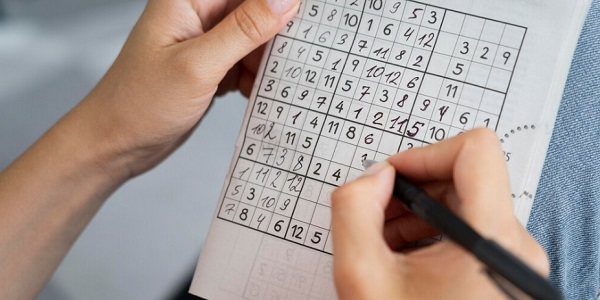# How to solve Sudoku for beginners and pros (part 2)

We continue to talk about the working techniques and ways of solving Sudoku used by champions.

The essence of the scheme is 1 column, 1 row and 1 square at a time. How does the technique work? Above we told you that we filled 2 squares using the "hanging fruit" method. The instructions were to find the easiest areas and fill them in.

Once we have entered the values into the squares, we move on. We need more information about what numbers to write where. The advantage of sudoku is the emergence of "new perspectives" as the box is filled in.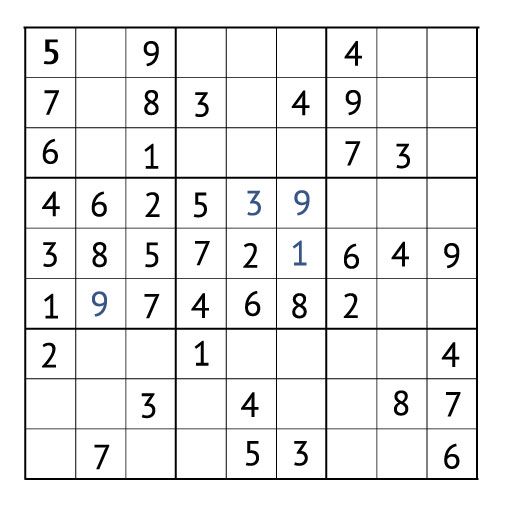Focus on one square

Let's see what we have going on in the centre row. Let's pay attention to the right column and the bottom row: the values 1, 9, 7, 4, 6, 8 and 2 are written here. By the method of elimination we understand that here we need a three and a five. We keep the information in our minds and look at the neighbouring squares. We need to analyse the numbers in them and see where we can insert 3 and 5. We look at the top right square. In the middle column there is a 3, i.e. it is already clear that 5 should be inserted here. The three is placed to the right of the five.

This solution simplifies the search for what values to write in the square. By the method of elimination we understand that there should be 1, 7 and 8. What exactly should we put in the free cells? Again we look at the squares at the top and bottom. There is space for 7 in the right and left columns. We place the seven in the middle column at the top.

So, we managed to place the numbers 3, 5 and 7. After that, the Sudoku game board looks like this.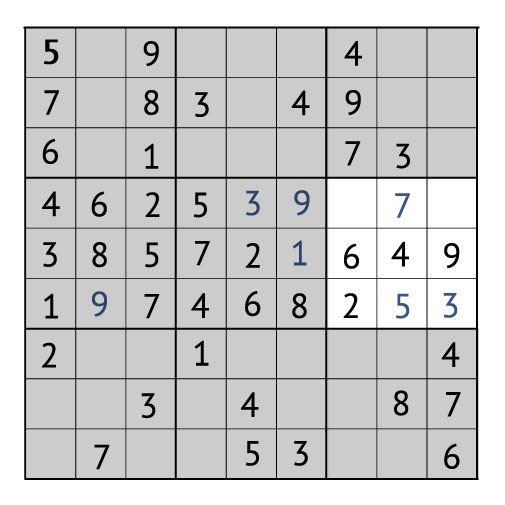The method of elimination in this case will not allow us to understand how to place the remaining values in the right centre square where 7, 6, 4, 9, 2, 5 3 are already inscribed. But let's see what happens when we place the first and second values in the square.

On the bottom right there is a square in which 8, 4, 7 and 6 are inscribed. Accordingly, we need to place 1, 2, 3, 5 and 9 in it. There are a lot of numbers. We need to figure out where they fit. To do this, we look at the neighbouring squares that are in the bottom row. Here it is necessary to look for intersections. A quick glance quickly clings to the triple, which is in both the first and second squares below. At the top right of the square we also have a 3. So, we can safely write the 3 in the upper left corner of the bottom right square.

It turns out that in the bottom right square we now have the value 3, 8, 4, 7 and 6. It remains to figure out how to place 1, 2, 5, 9. Let's see how things are with 5. The number 5 is found both in the middle square on the right (the middle column in the bottom row) and in the middle square at the bottom. Accordingly, we don't have so much choice. Five is asked under the number 3 (next to seven and eight).

So we already have 3, 5, 8, 4, 7 and 6 in the square. It remains to attach 1, 2 and 9. Let's see how things are in the upper rows of the squares on the left and in the centre. You can see that the top row of the bottom square is asking for a nine. This will allow us to finish the square quickly: we need to figure out where to put 1 and 2. It is immediately obvious that the two is in the left column in the right middle square. So, we only have to write a one. By the method of elimination we understand where to insert the two. I.e. a few details in the grid give us a hint on what to do next.

What we have done, what to do next

We look at the middle right square, which depends on the bottom square. We can see that the left column can be filled in, since it already contains 8 of the 9 digits (these are 1, 2, 3, 4, 5, 6, 7 and 9). Accordingly, only 8 are missing. To solve Sudoku puzzle, we write the number 8 and then the number 1 in the middle right square. This is how the game board turns out.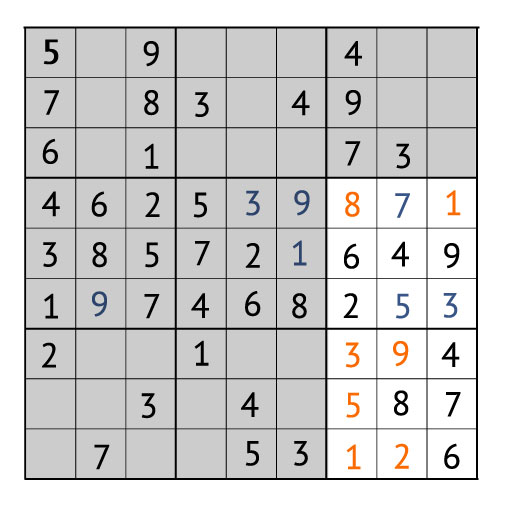We clung to columns, lines and squares, gradually filling them in. The result so far is that 4 of the 9 squares already contain numbers. We just have to work through the remaining 5. In the next tutorial, we will share some valuable know-how on how to do this quickly and easily.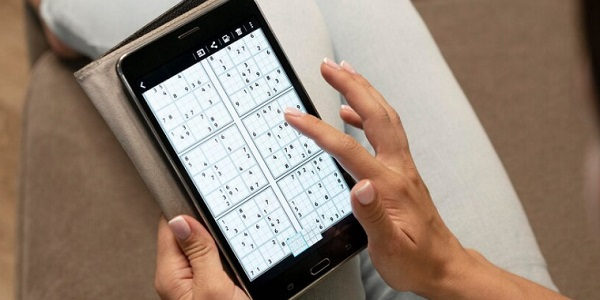##### 6 reasons to play Sudoku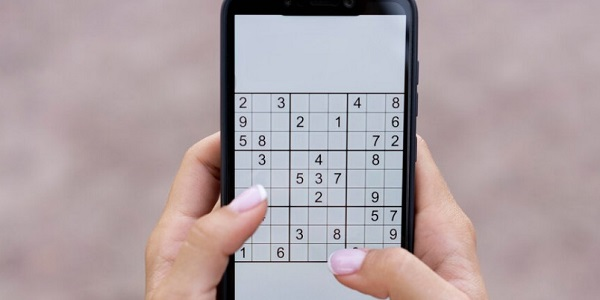##### Sudoku History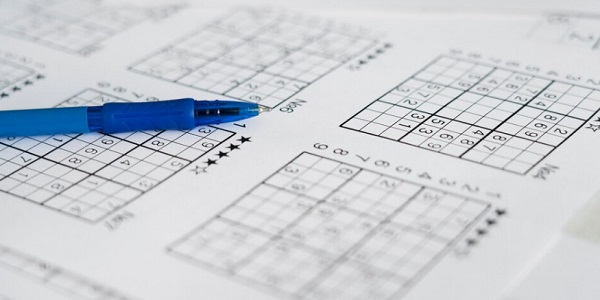##### 5 Sudoku solving tips for beginners